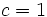# Finite Lazard Lie group

This page describes a group property obtained as a conjunction (AND) of two (or more) more fundamental group properties: finite group and Lazard Lie group
View other group property conjunctions OR view all group properties

## Definition

A finite Lazard Lie group is defined as a group$G$ satisfying the following equivalent conditions:

No. Shorthand Explanation
1 finite and a Lazard Lie group$G$ is a finite group and there is a natural number$c$ such that the 3-local nilpotency class of$G$ is at most$c$ and the order of$G$ does not have any prime divisors less than or equal to$c$.
2 product of its Sylow subgroups, each of which is a Lazard Lie group$G$ is a finite nilpotent group, i.e., it is the internal direct product of its Sylow subgroups, and each of its Sylow subgroups is a Lazard Lie group. Here, a finite$p$-group is a Lazard Lie group if its 3-local nilpotency class is at most$p$.

## Relation with other properties

### Stronger properties

Property Meaning Proof of implication Proof of strictness (reverse implication failure) Intermediate notions
finite abelian group any two elements commute Special case$c = 1$ |FULL LIST, MORE INFO
odd-order class two group (also called finite Baer Lie group) odd-order group of class at most two Special case$c = 2$ |FULL LIST, MORE INFO

### Weaker properties

Property Meaning Proof of implication Proof of strictness (reverse implication failure) Intermediate notions
finite nilpotent group Finite group that is 1-isomorphic to an abelian group|FULL LIST, MORE INFO